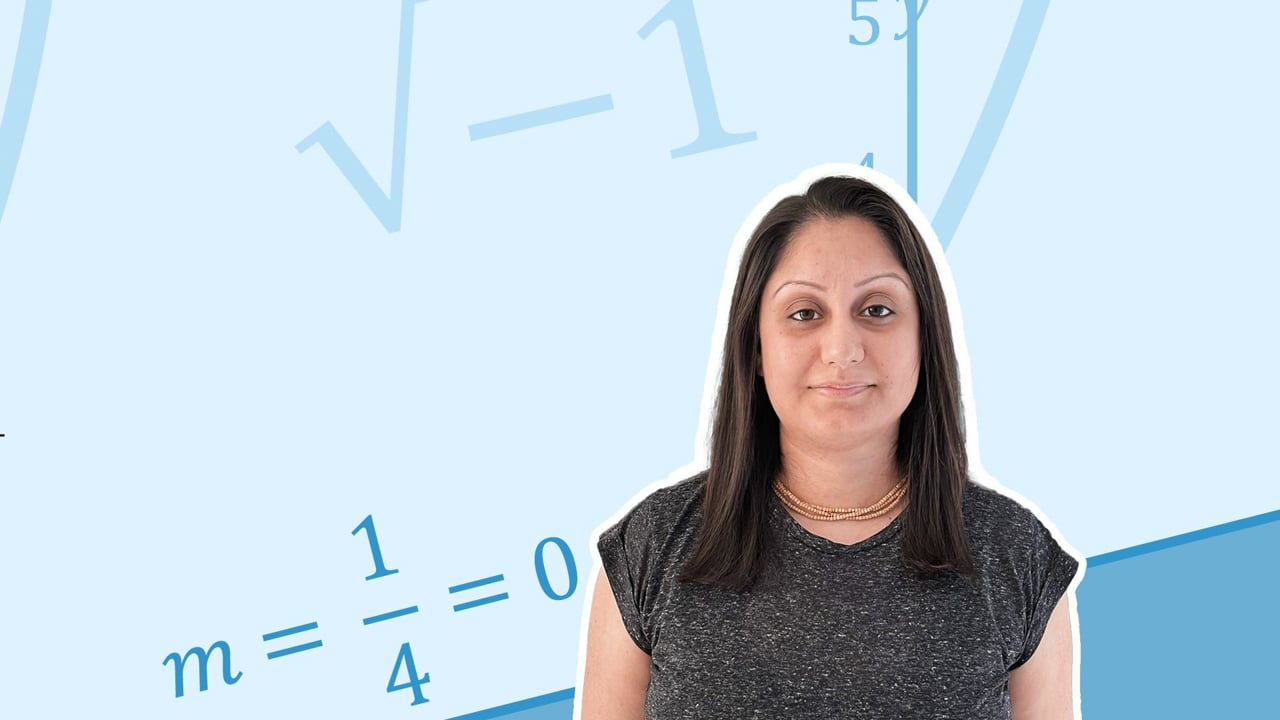Chapter overviewMaths

Exam board

AQA

Number

Algebra

Graphs

Ratio proportion and rates of change

Shapes and area

Angles and geometry

Trigonometry

Probability

Statistics

Maths

# Solving equations0%

Summary

# Solving equations

## ​​In a nutshell

An equation can be solved by rearranging. You usually want to find the value of the unknown variable, e.g. $x$. which makes the equation true. Think of an equation as a balancing scale, whatever you do to one side of the equation, you must do the same to the other.

## Balancing scales

$x+5=13$

can be represented by

To solve the equation for $x$, you need to rearrange the equation so that $x$ is by itself on one side of the equation, and all other numbers or terms should be on the other side. To get $x$ by itself, $+5$ needs to be moved to the other side. To do this, think of the inverse operation, or the opposite of $+5$. The opposite of $+5$ is $-5$ so subtract $5$ from both sides of the equation. This gives​

\begin {aligned} x &= 13 -5 \\ x &=\underline{8} \end {aligned}​​

This can be represented by

## Solving algebraically

When solving an equation, think about what to do to both sides of the equation to get the unknown variable by itself.

##### Example 1

\begin {aligned}\qquad x + 3 &= 7 \\-3 \qquad & \qquad -3\end {aligned}\\\quad \quad\underline {x=4}​​

When there is more than one number to move to the other side of the equation, it is important to move them in the right order. Use reverse BIDMAS to help.

##### Example 2

Solve the equation

$3x+4=10$

Here, the $3$ with the $x$ and $+4$ need to be moved to the other side. To decide which number to move across first, think about substituting a number in for $x$. You would take the value of $x$, multiply by $3$ first, then $+4$ to the result. So when solving an equation, reverse the process. So move $+4$ to the other side first, then move $3$ to the other side.

The opposite of $+4$ is $-4$, so subtract $4$ from both sides. Then $3$ is multiplying $x$, so do the inverse and divide by $3$ to both sides.

\begin {aligned}\qquad 3x+4 &=10 \\-4 \qquad & \qquad -4 \\3x &= 6 \\\div3 \qquad & \qquad \div 3 \end {aligned}\\\quad \quad\underline {x=2}

##### Example 3

Solve

$3x+32 = 11x-48$​​

When there are multiple terms with $x$ in the equation, on both sides, first move the $x$ terms on one side, and the numbers onto the other side. Move the $x$'s onto the side where there are more of them.​

\begin {aligned}\quad 3x+32 &= 11x -48 \\-3x \qquad & \qquad -3x \\32 &= 8x - 48 \\+48 \qquad & \qquad +48 \\80 &= 8x \\\div 8 \qquad & \qquad \div 8 \\10 &= x \end {aligned}\\\quad \underline {x=10}